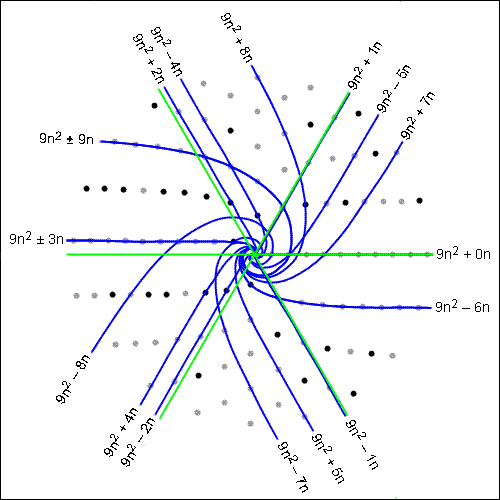NumberSpiral.com

CONTENTSEvery offset curve can be generated by a quadratic function of the form: y = ax2 + bx + c Where y is the number that appears on the graph; a, b, and c are constants that define the curve; and x is the index of the integer on the curve. In order for a quadratic function to be an offset curve, a must be a perfect square. In order for a function to be a composite offset curve, c must be zero. In other words, the function for a composite offset curve looks like this: y = ax2 + bx Of course a must be a perfect square in the above equation, because composite curves are a subset of offset curves. For composite offset curves of angle n/d where the denominator is even: a = (d/2)2 b = n offset = (n/d)2 For angles with odd denominators, multiply the numerator and denominator by two and use the above formulas. The correspondence between angles and coefficients creates an orderly pattern on the graph. This can be seen in the following illustration which shows all the composite curves for denominator = 6 with the numerator ranging from –9 to 9 inclusive:VortexTo draw these curves with Vortex, use the coe command to enter coefficients. For example, to show 9n2 + 3n you would enter "coe 9 3 0". The printed expressions will not appear in Vortex.
Figure 1

 Parameters of the curves in Figure 1 are shown in the following table.b Angle Offset Factorization –9 –9/6 81/36 x(9x–9) –8 –8/6 64/36 x(9x–8) –7 –7/6 49/36 x(9x–7) –6 –-6/6 36/36 x(9x–6) –5 –5/6 25/36 x(9x–5) –4 –4/6 16/36 x(9x–4) –3 –3/6 9/36 x(9x–3) –2 –2/6 4/36 x(9x–2) –1 –1/6 1/36 x(9x–1) 0 0/6 0/36 x(9x) 1 1/6 1/36 x(9x+1) 2 2/6 4/36 x(9x+2) 3 3/6 9/36 x(9x+3) 4 4/6 16/36 x(9x+4) 5 5/6 25/36 x(9x+5) 6 6/6 36/36 x(9x+6) 7 7/6 49/36 x(9x+7) 8 8/6 64/36 x(9x+8) 9 9/6 81/36 x(9x+9)1  2  3  4  5  6  7  8  9  10   Next